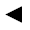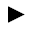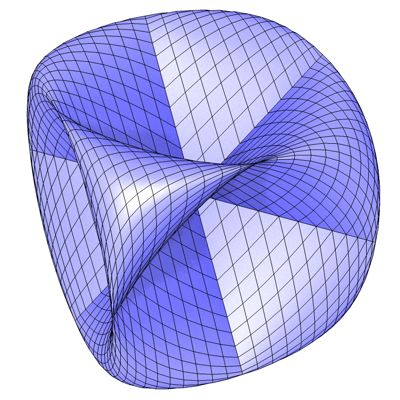[back]

## 3.16. Sine Surface

[next]The Sine Surface [ 6,11 ] is represented by the following equations.

 x = sin(u) 3-62 y = sin(v) 3-63 z = sin(u + v) 3-64

The sine surface is the counterpart to the cosine surface .

To represent the area, the two parameters u and v must have the following values ​​(definition range).

 u is an element from the number set [-pi, pi] v is an element from the number set [-pi, pi]Fig. 20